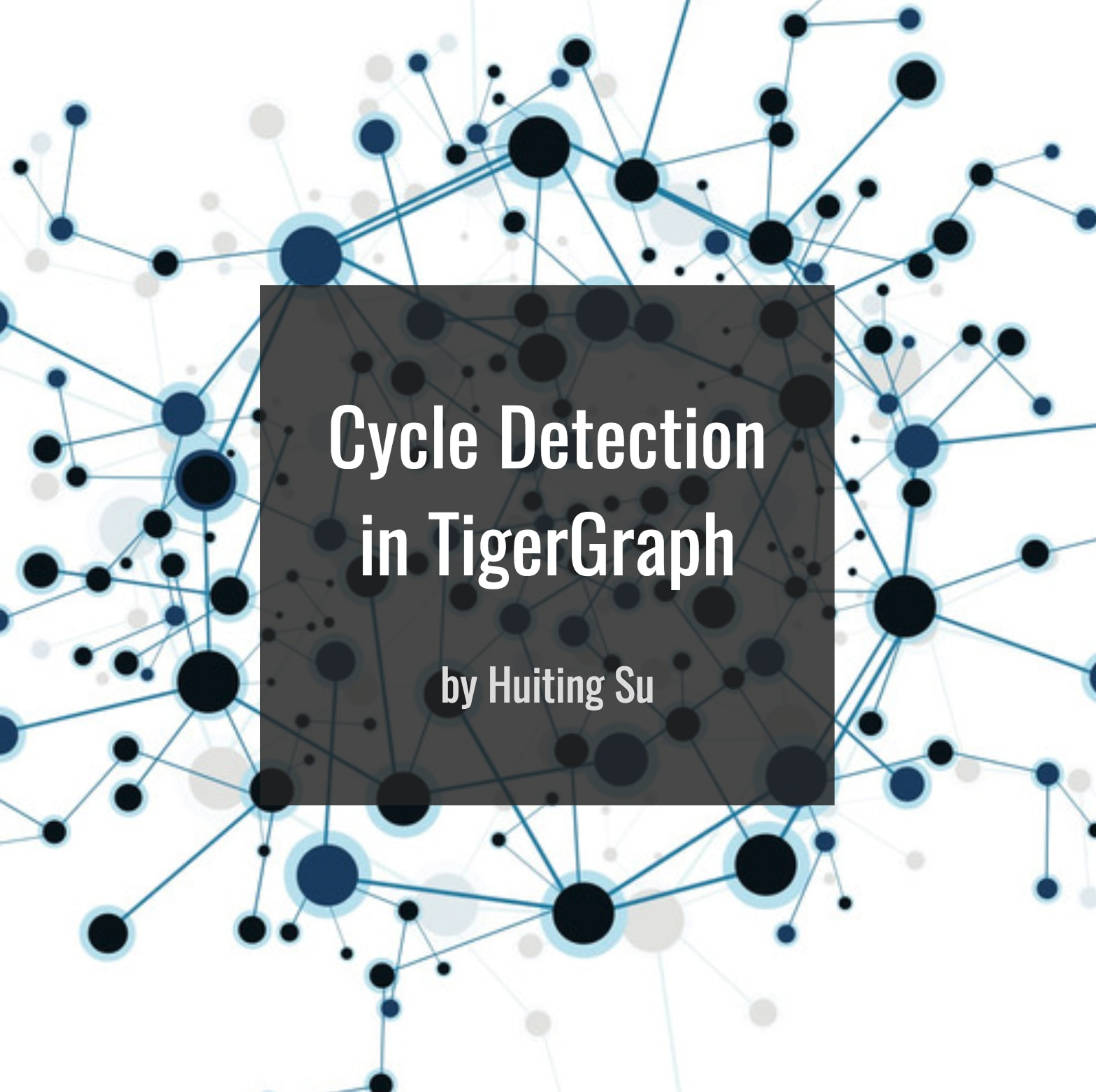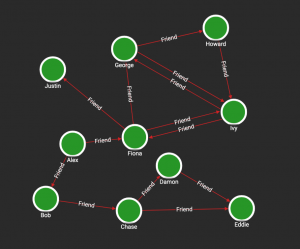# Cycle Detection in TigerGraph## 09 May Cycle Detection in TigerGraph

A cycle is a path of edges and vertices that connect together to form a loop. In a directed graph, all the edges must point in the same direction so that one can “travel” around the cycle. In a sample social graph (Fig.1), George, Howard and Ivy form a cycle, but Chase, Damon and Eddie do not.Fig.1 Sample Social Graph

A classic problem in graph theory is directed cycle detection, finding and reporting all the cycles in a directed graph. This has important real-world applications, for money laundering and other fraud detection, feedback control system analysis, and conflict-of-interest analysis. Cycle detection is often solved using Depth First Search, however, in large-scale graphs, we need more efficient algorithms to perform this.

The Rocha-Thatte algorithm is a distributed algorithm, which detects all the cycles in a directed graph by iteratively having each vertex update and forward path traversal sequences to its out-neighbors. In this short technical blog I will show you how to implement the Rocha-Thatte algorithm in GSQL, and how it can efficiently be used for cycle detection.

Each vertex has a local accumulator to store its current set of sequences of vertex ids.  Initially, each vertex starts with one sequence, containing its own id. In every iteration, the vertices send their sequences to their out-neighbors. Meanwhile, they receive sequences from their in-neighbors and append their own ids to each received sequence.

When the current vertex is the same as the first vertex in a sequence, a cycle is detected. For example, in the sample social graph, when vertex “Ivy” receives a sequence of [“Ivy”, “George”, “Howard”], we detect a cycle. We stop passing that sequence, and want to report that cycle. However, every cycle is detected by all vertices in that cycle in the same iteration. Because every vertex is a starting point and is also passing messages, we would find not only [“Ivy”, “George”, “Howard”, “Ivy”], but also [“George”, “Howard”, “Ivy”, “George”] and [“Howard”, “Ivy”, “George”, “Howard”]. To avoid duplicates, it is reported only by the vertex with the “minimum” label in the sequence. Any consistent method for picking the minimum label is okay. An obvious choice here would be alphabetical sorting (so “George” is first), but for efficiency and to work with any vertex type, we use our database’s internal numeric ids.

There is another case where the sequence contains the current vertex, but the current vertex is not the first one in the sequence. For example, vertex “George” receives a sequence of [“Fiona”, “George”, “Howard”, “Ivy”]. In this case, a cycle is detected, but we do not report it since this cycle must have already been reported in an earlier iteration. For example, it took 4 iterations to find this cycle, but it took only 3 iterations starting from George; these non-starting-point cycles are only making discoveries that were already made before. Thus, the sequence is discarded.

If any vertex did not receive messages in the previous iteration, it is deactivated. The algorithm stops when all the vertices in the graph are deactivated.

GSQL handles distributed algorithms like Rocha-Thatte cycle detection very easily. A sample query which performs this algorithm is shown below:

``````CREATE QUERY cycle_detection (INT depth) FOR GRAPH social {
ListAccum<ListAccum<VERTEX>> @currList, @newList;
ListAccum<ListAccum<VERTEX>> @@cycles;
SumAccum<INT> @uid;

# initialization
Active = {Person.*};
Active = SELECT s
FROM Active:s
ACCUM s.@currList = [s];

WHILE Active.size() > 0 LIMIT depth DO
Active = SELECT t
FROM Active:s -(Friend:e)-> :t
ACCUM BOOL t_is_min = TRUE,
FOREACH sequence IN s.@currList DO
IF t == sequence.get(0) THEN  # cycle detected
FOREACH v IN sequence DO
IF getvid(v) < getvid(t) THEN
t_is_min = FALSE,
BREAK
END
END,
IF t_is_min == TRUE THEN  # if it has the minimal label in the list, report
@@cycles += sequence
END
ELSE IF sequence.contains(t) == FALSE THEN   # discard the sequences containing t
t.@newList += [sequence + [t]]   # store sequences in @newList to avoid confliction with @currList
END
END
POST-ACCUM s.@currList.clear(),
t.@currList = t.@newList,
t.@newList.clear()
HAVING t.@currList.size() > 0;  # IF receive no sequences, deactivate it;
END;
PRINT @@cycles;
}
``````

If we run this query on the social graph shown in Figure 1, we will get the result below.

JSON result:

``````[
{
"@@cycles": [
[
"Fiona",
"Ivy"
],
[
"George",
"Ivy"
],
[
"Fiona",
"George",
"Ivy"
],
[
"George",
"Howard",
"Ivy"
],
[
"Fiona",
"George",
"Howard",
"Ivy"
]
]
}
]
``````

Note that I use two accumulators called @currList and @newList. In every iteration, every vertex sends and receives the sequences at the same time. It is like having some outgoing mail in the mailbox, and one mail carrier gathers the mail from the mailbox, while another mail carrier puts incoming mail into the mailbox — if the two do not synchronize their efforts correctly, the system will not work. We need to separate the outgoing mail and incoming mail in two mailboxes, namely @currList and @newList.  This algorithm is efficient in TigerGraph because it computes for the vertices in the same iteration in parallel.

, ,